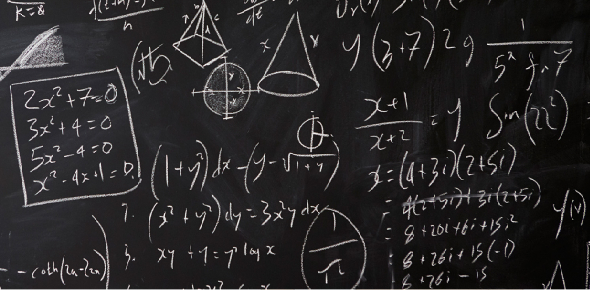10 QuestionsSettingsRelated Topics
• 1.
• 2.
This number line shows an integer addition equation. What is the equation that this number line shows?
• A.

2 + 7 = 9

• B.

-5 + 7 = 2

• C.

-5 + 2 = 7

• D.

-7 + 5 = -2

• 3.
• 4.
Solve by adding these integers: 4 + (-5)
• 5.
This number line shows an integer addition equation. What is the equation that this number line shows?
• A.

-2 + (-9) = 6

• B.

-2 + 6 = -9

• C.

6 + (-9) = -2

• D.

6 + (-2) = -9

• 6.
• 7.
• 8.
• 9.
CAHSEE Sample problem: Which of the following numerical expressions results in a negative number?
• A.

(–7) + (–3)

• B.

(–3) + (7)

• C.

(3) + (7)

• D.

(3) + (–7) + (11)